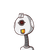# 12 articles each weighing 250grams and 8 articles each weighing 300 grams are packed in box the weight of box along with articles

12 articles each weighing 250grams and 8 articles each weighing 300 grams are packed in box the weight of box along with articles is 6 kg 400 gram what is weight of empty box

### 1 thought on “12 articles each weighing 250grams and 8 articles each weighing 300 grams are packed in box the weight of box along with articles”

1.1) Empty box weight = 1 kg.or 1,000g.

### Given :

1).12Articles each weight is 250 grams .

2)8 articles each weight is 300grams.

Those articles are packed in a box weight is = 6 kg 400 g.

### To find:

1) Find the weight of the empty box .

Step-by-step explanation:

1)12articles each weight is 250grams .

12×250= 3,000g.

Then total weight is =3,000 grams

Therefore 3,000 grams was convert into kg= 3 kg.

2) 8 articles each weight is 300grams.

8 × 300 = 2,400g.

Then total weight is =2,400.

Therefore 2,400g was convert into kg = 2 kg 400 grams .

### since two articles weight was make addition.

3,000+ 2,400=5,400 gram this is the total weight of the article.

so we make addition with3,000 + 2,400 = 5 kg 400g .

### Therefore we make substraction between those articles weight and total parcel weight.

*5,400-6,400=1,000 because 1,000means 1 kg it is the weight of the empty box.